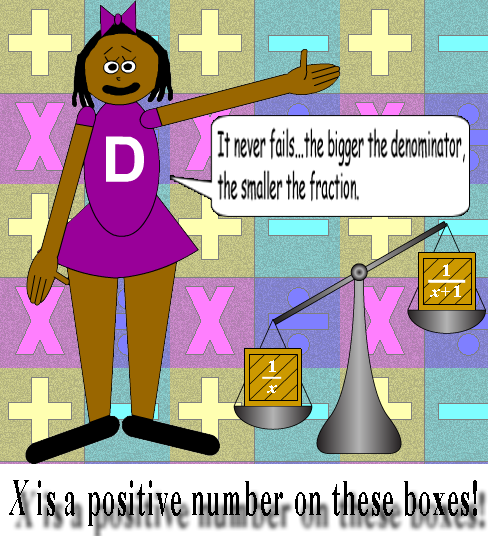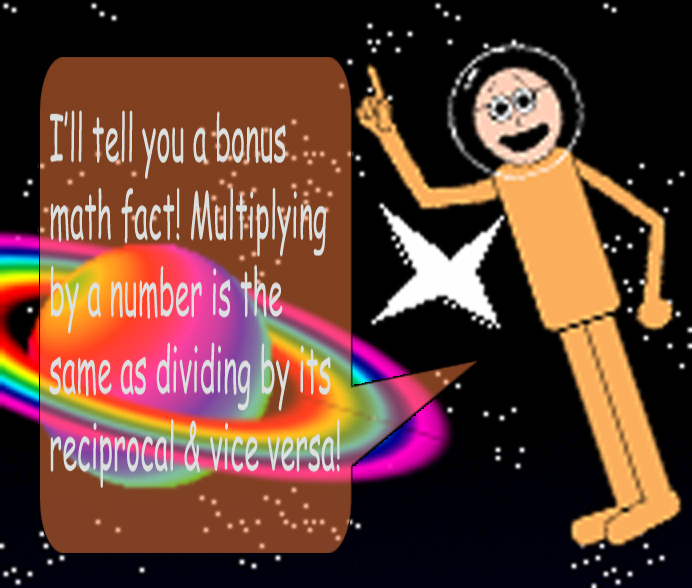# Unit Fraction Uniqueness 3

## When you divide unit fractions with consecutive denominators, something quite interesting happens! Can you figure out the pattern to the table below? The last row will show you the formula!

Fraction #1Fraction #2 Quotient
1/21/3 3/2 or 1 + 1/2
1/31/4 4/3 or 1 + 1/3
1/41/5 5/4 or 1 + 1/4
1/51/6 6/5 or 1 + 1/5
1/61/7 7/6 or 1 + 1/6
1/71/8 8/7 or 1 + 1/7
1/81/9 9/8 or 1 + 1/8
1/91/10 10/9 or 1 + 1/9
1/x1/(x+1) (x+1)/x or 1 + 1/x

## (x + 1)/x = 1 + 1/x. (x ≠ 0)

### Division is NOT commutative, so if you swap Fractions #1 & #2, then...

Fraction #1Fraction #2Quotient
1/31/2 2/3
1/41/3 3/4
1/51/4 4/5
1/61/5 5/6
1/71/6 6/7
1/81/7 7/8
1/91/8 8/9
1/101/9 9/10
1/(x+1)1/x x/(x+1)

## The denominator of the 2nd fraction becomes the numerator in the quotient & the denominator of the 1st fraction becomes the denominator in the quotient!

However, there's only 1 difference this time: the quotient is less than 1. That's because the smaller fraction gets divided by the bigger fraction. The quotients in the 2nd table are the reciprocals of the quotients in the 1st table!### Finally, when you divide by a fraction, you're also multiplying by its reciprocal!